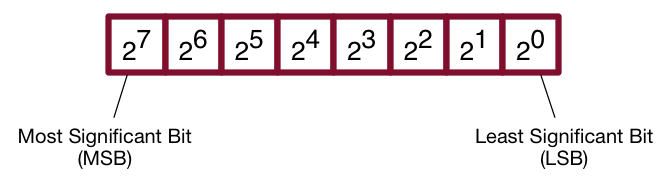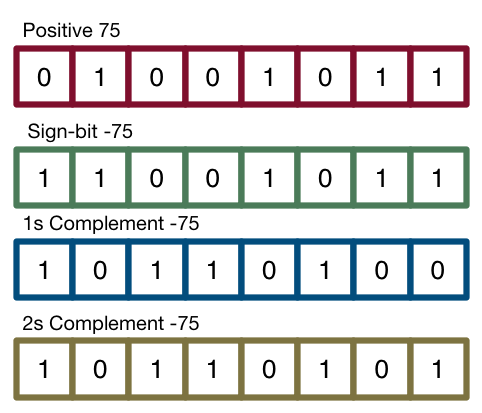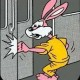# Representing Numbers in Binary

Before we can really start writing interesting programs with our ARM, even simple ones, we need to understand a bit about how how a computer actually works with numbers.

When people talk about computers, they frequently say something like “It’s all zeros and ones”. That’s wrong, in a couple of different ways.

First, when you look at the actual computer hardware, there are no zeros and ones. There are two kinds of signals, represented as two different voltage levels. You can call them “high” and “low”, or “A” and “B”, or “+” and “-“. It doesn’t matter: it’s just two signals. (In fact, one fascinating thing to realize is that there’s a fundamental symmetry in those signals: you can swap the choice of which signal is 0 and 1, and if you just swap the and gates and the or gates, everything you won’t be able to tell the difference! So one ARM processor could use one signal for 1, and a different ARM could use that signal as 0, and you wouldn’t actually be able to tell. In fact, everyone does it the same way, because chip design and manufacture are standardized. But mathematically, it’s possible. We’ll talk about that duality/symmetry in another post.)

Second, the computer really doesn’t work with numbers at all. Computer hardware is all about binary logic. Even if you abstract from different voltages to the basic pairs of values, the computer still doesn’t understand numbers. You’ve got bits, but to get from bits to numbers, you need to decide on a meaning for the bits two possible values, and you need to decide how to put them together.

That’s what we’re really going to focus on in this post. Once you’ve decided to call on of the two primitive values 1, and the other one 0, you need to decide how to combine multiple zeros and ones to make a number.

It might seem silly, because isn’t it obvious that it should use binary? But the choice isn’t so clear. There have been a lot of different numeric representations. For one example, many of IBM’s mainframe computers used (and continue to use) something called binary coded decimal (BCD). It’s not just different for the sake of being different: in fact, for financial applications, BCD really does have some major advantages! Even if you have decided to use simple binary, it’s not that simple. Positive integers are easy. But how do you handle negative numbers? How do you handle things that aren’t integers?

We’re going to start with the simplest case: unsigned integers. (We’ll worry about fractions, decimals, and floating point in another post.) Like pretty much all modern computers, the ARM uses the basic, mathematical binary exponential representation. This works basically the same as our usual base-10 numbers. We look at the digits from right to left. The leftmost digit (also called the least significant digit counts ones; the next digit counts 10s; the next counts 100s, and soon. So in base 10, the number 3256 means 6*100 plus 5*101 plus 2*102 plus 3*103.In binary, we do exactly the same thing, only we do it with powers of 2 instead of powers of 10. So in binary the number 1001101 is 1 + 0*21 + 1*22 + 1*23 + 0*24 + 0*25 + 1*26 =1 + 4 + 8 + 64 = 77.

Arithmetic on binary is easy enough – you do the same thing you would with decimal, but in subtraction, borrows give you 2, not 10. As a quick example, let’s look at 7 + 13, which is 111 + 1101.

1. We start at the right edge. We have 1 + 1 = 10 – so the first digit of the sum is 0, and we carry 1.
2. Next we have 1 + 0 + 1(carry) = 10 – so the second digit is again 0, and we carry 1. Our sum so far is 00.
3. Now we’re on to the third digit. 1 + 1 + 1(carry) = 11. So the third digit is 1, and we carry one. Our sum so far is 100.
4. Now the fourth digit, 1 + 0 + 1(carry), so we get 10. So the sum is 10100, or 20.

We’ll ignore subtraction for a moment, because as we’ll see in a little while, in computer hardware, we don’t actually need to have subtraction as a primitive. Addition is the core of what computer arithmetic, and we’ll use addition to implement subtraction by taking the negative of the number being subtracted. (That is, to compute A-B, we’ll do A+(-B).)

Positive integers with addition aren’t enough to do most stuff we want to do on a computer. Even if we’re never going to write a program that manipulates numbers, we absolutely need to be able to subtract. To a computer, the only way to compare values is to subtract one from another! So we need to be able to do negatives and subtractions. How can we represent negative numbers in binary?

There are three basic choices, called sign-bit/sign-magnitude, one’s-complement and two’s complement. I’ve put an example of the three representing the number 75 in the figure below.In sign-bit representation, what you do is take the leftmost bit (also called the high-order bit), and use it to indicate sign (for obvious reasons, you call it the sign bit.). If the sign bit is 0, then the number is positive; if it’s 1, then the number is negative. For example, 01010 would be +10; 11010 would be -10.

For a human being, sign-bit looks great. But in practice, sign-bit was never used much, because while it looks simple to a human, it’s quite complicated to build in computer hardware. IBM did use it in some early machines, but even they gave up on it.

Next is one’s complement. In 1’s complement, high order bit is still a sign bit. But to convert a number from positive to negative, you don’t just change the sign bit – you invert every single bit in the number. You can still tell whether a number is positive or negative by its sign bit, but the rest of the bits are also different. +10 in one’s complement binary is 01010; -10 is 10101.

Arithmetic in 1s complement is a bit weird. You can almost just add a negative number to a positive number as if they were both positive. Almost, but not quite.

For example, let’s try 6 + -6. 6 is 0110, and -6 is 1001. Add them up: 1111. In twos complement, that’s -0. And there’s one of the weird issues about one’s complement: it’s got two distinct values for 0 – +0 and -0. Since they’re both just 0, we treat them as equal, and it’s not really a problem.

How about 6 + -8? 6 is 00110 (we need 5 bits to handle 8), and -8 is 10111. Add
them up, and you get 11101 – which is -2, the correct answer.

Now, what about 8 + -6? 8 is 01000, and -6 is 11001. Add them up, and you
get 00001, with a carry of 1. So 8 + -6 = 1? That’s wrong! In one’s complement,
there are a bunch of places where simple binary addition will be off by one.
So you need to work out the algorithm for where it’s off-by-one and where it’s not, and you need to build more complicated hardware to incorporate it. That’s not attractive.

Still, one’s complement has been used a lot. In particular, one of the first computers I got to use was an old, beaten-up PDP-1, which used 1’s complement numbers.

Finally, we get to the representation that’s used in pretty much all modern computers: 2’s complement!

Once again, 2’s complement uses a sign bit. But instead of flipping all of the bits, you do something different. In 2’s complement, you need to know how many bits you’re using. If you’re doing an N-bit 2’s complement binary number, then the number -x is represented by 2N-x.

So if we’re doing 6 bits, and we wanted to represent -5, then we’d take 26-5, or 64-5=59. In binary, that’s 111011.

The really beautiful thing about 2s complement is that it’s pretty much the same thing as a trucated 2-adic integer – which means that arithmetic just works. If you’re adding two numbers, it doesn’t matter whether they’re signed numbers or not – it works.

It’s also really easy to implement negation. You don’t have to do that whole “subtract from 2^N” thing. In 2s complement, -N is 1+(ones_complement(N)). That’s super-easy to implement in hardware, and it’s also easy to understand and do for a human: flip the bits and add one!

Two’s complement is, in my opinion, a clear winner in integer representation, and the world of computer hardware maker agrees – everyone now uses 2’s complement for integers.

## 7 thoughts on “Representing Numbers in Binary”

1.John Armstrong

I’m curious if any processors have used a Gray representation.

1.medivh

John, as far as I’m aware, there’s a lot of applications for Gray code in microcontrollers on sensors. Angular sensors in particular, I’m told, but any application where the sensor reading value tends to go in one direction and needs reporting at very short intervals.

Then again, I’ve been wrong before and this is off the top of my head. So, grain of salt.

2.Johnny Maltby

“The leftmost digit (also called the least significant digit counts ones”

Not to be nitpicky, but I think this should be the rightmost digit.

Anyways, thanks for your posts, I am learning a ton from you!

3.JoeDude

John Armstrong, you’re needed as a mathematician to formalize some math using the Isabelle theorem assistant, work that would be specialized towards the way mathematicians work. So far, it’s dominated by computer scientists, since that’s who’s developed it.

Don’t get me wrong, though, that the computer scientists aren’t doing some classical-style math with it (though using types). The foundation, in many ways, models classical math, because of the influence of Larry Paulson, who got his B.S. in math at CalTech. The developers also do a lot of math with it, to the extent that I thought initially it was being developed in a math department.

Do a search on “Isabelle , Cambridge, TUM”. The learning curve is big, but it’s on the verge of going big time with the masses. If you don’t go for Isabelle, you’ll still eventually hear about it.

If you don’t go for it, that’s just more opportunity for people like me, who only have a B.S. in math.

4.Alex Vincent

Ahh, nice. You mention you’ll write about fractions & floating point in a secondary post. I’d really appreciate it if you could another post beyond that about modeling other types of numbers (BigDecimal, irrational numbers, numbers represented by an infinite series, complex numbers, etc.) in C++, and the relative costs of doing so. It’s a subject I’ve wondered about for years.

5.Felpe
1.markcc Post author